### Linear Test

Consider the linear model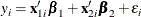where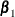and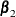are
and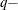dimensional unknown parameters, and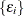,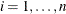, are errors with unknown density function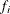. Let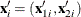;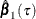and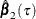be the parameter estimates forandrespectively at thequantile. The covariance matrix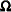for the parameter estimates is partitioned correspondingly as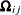with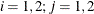; and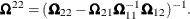#### Testing Effects of Covariates

Three tests are available in the QUANTREG procedure for the linear null hypothesis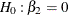at thequantile.

The Wald test statistic, which is based on the estimated coefficients for the unrestricted model, is given by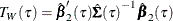where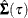is an estimator of the covariance of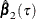. The QUANTREG procedure provides two estimators for the covariance, as described in the previous section. The estimator based on the asymptotic covariance is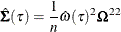where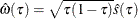and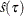is the estimated sparsity function. The estimator based on the bootstrap covariance is the empirical covariance of the MCMB samples.

The likelihood ratio test is based on the difference between the objective function values in the restricted and unrestricted models. Let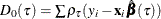and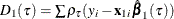, and set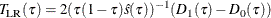whereis the estimated sparsity function.

The rank test statistic is given by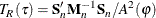where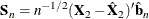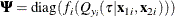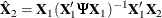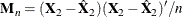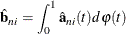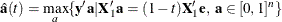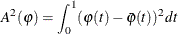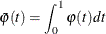and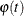is a score function.

The following score functions are available in the QUANTREG procedure:

Wilcoxon scores: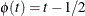Normal scores: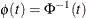, where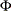is the normal distribution function

Sign scores: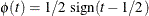Tau scores: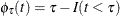.

The rank test statistic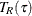, unlike Wald tests or likelihood ratio tests, requires no estimation of the nuisance parameterunder iid error models (Gutenbrunner et al., 1993).

Koenker and Machado (1999) prove that the three test statistics (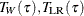, and) are asymptotically equivalent and that their distributions converge to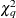under the null hypothesis, where q is the dimension of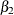.

#### Testing for Heteroscedasticity

After you obtain the parameter estimates for several quantiles specified in the MODEL statement, you can test whether there are significant difference for the estimates for the same covariates across the quantiles. For example, if you want to test whether the parametersare the same across quantiles, the null hypothesis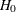can be written as: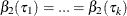, where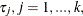are the quantiles specified in the MODEL statement. See Koenker and Bassett (1982a) for details.